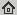# Techniques for gradient based bilevel optimization with nonsmooth lower level problems

Journal of Mathematical Imaging and Vision, 56(2): 175-194, Oct 2016
Abstract: We propose techniques for approximating bilevel optimization problems with non-smooth and non-unique lower level problems. The key is the substitution of the lower level minimization problem with an iterative algorithm that is guaranteed to converge to a minimizer of the problem. Using suitable non-linear proximal distance functions, the update mappings of such an iterative algorithm can be differentiable, notwithstanding the fact that the minimization problem is non-smooth. This technique for smoothly approximating the solution map of the lower level problem raises several questions that are discussed in this paper.

## BibTex reference

```@Article{OB16,
author       = "P. Ochs and R. Ranftl and T. Brox and T. Pock",
title        = "Techniques for gradient based bilevel optimization with nonsmooth lower level problems",
journal      = "Journal of Mathematical Imaging and Vision",
number       = "2",
volume       = "56",
pages        = "175-194",
month        = "Oct",
year         = "2016",
url          = "http://lmb.informatik.uni-freiburg.de/Publications/2016/OB16"
}
```